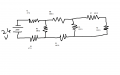#### Nathan Hale

Joined Oct 28, 2011
159
Hello Everyone, I am new to this forum and glad i found it.
I was wondering if you guys knew of any websites which talk about a ladder network. I really want to get to know how a ladder network works.I am also attaching a picture of the network. It would be nice if some one told me how much current will be flowing through R4 and R3. Please let me know how you got that answer.Hoping on asking more questions later.
Thank You

#### JoeJester

Joined Apr 26, 2005
4,390
The proceedure would be in Volume 1 above.

•Nathan Hale

#### jimkeith

Joined Oct 26, 2011
540
This is how I solved it--did not recheck the math...
First reposition series elements to simplify--current is equal in all parts of series circuit
Separate out the first attenuator
Calculate the thevenin open circuit voltage and equivalent series resistance
(short voltage source and put both resistor legs in parallel)--Requiv
Calc the new thevenin Eoc & Requiv
Solve for current (I = E/R)

There may be a better way as there are always multiple methods

#### Attachments

Last edited:
•Nathan Hale

#### t_n_k

Joined Mar 6, 2009
5,455
As you say there are several options for solving.

My approach would be

1. Find the total resistance seen by the 24V source [Rtot=166.7273Ω]
2. Find current from 24V source [Isource=24/Rtot=0.1439477A]
3. Recall the equivalent resistance of the network part comprising R4 to R8 [R48=266.667Ω]
4. Use current divider rule to find currents in R3 & R4 shown in the following steps 5 & 6
5. I_R3=Isource*R48/(R3+R48)= ??
6. I_R4=Isource*R3/(R3+R48)= ??

•Nathan Hale